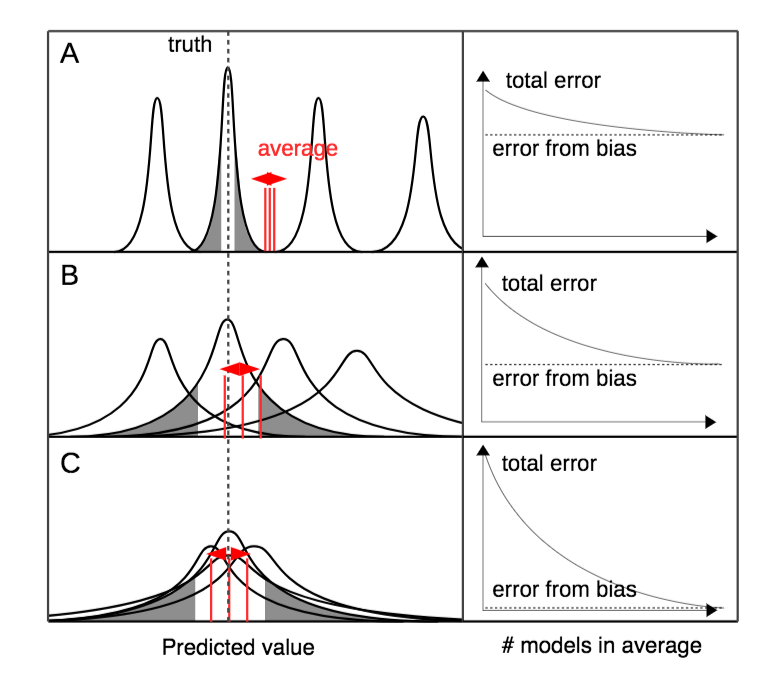Papers

# Model averaging in ecology: a review of Bayesian, information-theoretic and tactical approaches for predictive inference

The review paper, by Dormann, C.F., Calabrese, J.M., Gurutzeta, G., Matechou, E., Bahn, V., Bartoń, K., et al. to appear in Ecological Monographs, explores different model averaging techniques in terms of ways to calculate the model weights and to combine predictions from different models. The advantages and disadvantages of model averaging are discussed and code for methods falling under three categories (Bayesian, information theoretical and tactical) is provided.

Read a blog post written by the main contributors of the paper here.

Standard
Uncategorized

# Diana gave talk at the University of Nottingham

On 25th April Diana gave a talk on Parameter Redundancy and Identifiability at the University of Nottingham as a joint seminar of the  Algebra and Analysis group and the Statistics and Probability group.

The abstract for the talk was:

Mathematical and statistical models can be used to describe different systems or provide a representation of a process, which are defined by parameters that describe different characteristics that underlie the model. For example, a dynamic system could be used to describe a pharmacokinetic system with a parameter representing the infusion rate of a drug or a statistical model could be used to describe an ecological population, with a parameter describing the survival probability of an animal.  Determining or estimating these parameters will provide key information about the underlying process, though it is not always possible to estimate every parameter.  Such a problem stems from the inherent structure of a model; for example two parameters could be confounded and only ever appear as a product. This is known as parameter redundancy or the parameters are termed unidentifiable.

It may not be obvious whether parameters in a model are identifiable. A general method for checking identifiability involves forming a matrix of derivatives and calculating its rank, which can be executed in symbolic algebra packages such as Maple. However for more structurally complex models Maple can run out of memory trying to calculate the rank. This talk discusses two methods to solve this problem. The first uses reparameterisation to simplify the derivative matrix and allow the calculation of the rank, and the second involves calculating the rank numerically.

Standard